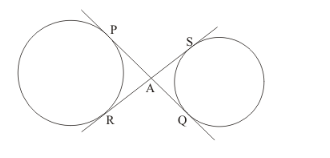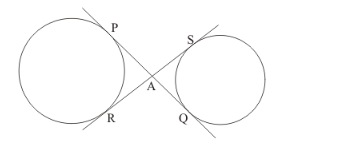# In the given figure, common tangents PQ and RS to

Question:

In the given figure, common tangents PQ and RS to two circles intersect at A. Prove that PQ = RS.Solution:

The figure given in the question isWe know from the property of tangents that the length of two tangents drawn from a common external point will be equal. Therefore,

PA = RA …… (1)

AQ = AS …… (2)

Let us add equation (1) and (2)

PA + AQ = RA + AS

PQ = RS

Thus we have proved that PQ = RS.